Study Guide

A quadratic equation is a polynomial of degree 2. It always has an x2 term, but no larger exponents. Another way to write that, with more letters but fewer words, is that a quadratic equation has the form y = ax2 + bx + c, where a ≠ 0.

Solving an equation means to find all values of x that, when plugged into the equation, will make the whole thing equal zero. That is, what values will make ax2 + bx + c = 0? It's like a puzzle, and you're looking for a five-letter word that means 'the solution to the equation.' Those values of x are the roots of the equation.

Sometimes, solving a quadratic equation is easy. Take x2 = 4, for instance. You can take the square root of both sides of the equation, finding x = 2 and x = -2 as the solutions. Other times, you can factor the quadratic equation into a squared term: x2 + 2x + 1 = 0 becomes (x + 1)2 = 0, giving a solution of x = -1.But quadratic equations don't always play nice. What can we do when they start getting unruly? We'll look at two techniques that can be used on any quadratic equation. We'll also go over a method for predicting the number and type of solutions you'll find for any particular equation. Buckle up.

### Sample Problem

Solve the quadratic equation x2 + 2x + 5 = 0.

Hmm, now this is tricky. This equation is—most definitely—not factorable. It would be really convenient if it were factorable, though; this is especially the case if it factored into a squared term. In fact, we can make it do just that, and that's what completing the square is all about.

Take another look at the equation above, this time with some parentheses thrown in.

(x2 + 2x) + 5 = 0

It looks like x2 + 2x would be factorable if we had another term in the parentheses. If we pick the right number, it would even factor into a squared term, (x + d)2. For this equation, that number is 1:

(x2 + 2x + 1) = (x + 1)2

We can even pull a little bit of algebraic wizardry to make it happen. Check it out: (+1 – 1) = 0. Who cares if we add zero to an equation? No one, that's who. We can add and subtract a 1 to the left side of our original equation without technically changing anything.

(x2 + 2x) + 5 (+ 1 – 1) = 0

(x2 + 2x + 1) + 5 – 1 = 0

(x + 1)2 + 4 = 0

Now that we have all of the x's inside the squared term, we can do this:

(x + 1)2 = -4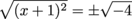x + 1 = ± 2i

x = -1 + 2i and x = -1 – 2i

Did we really just solve an unfactorable equation? Let's check our answers and make sure.

x2 + 2x + 5 = 0

(-1 + 2i)2 + 2(-1 + 2i) + 5

(1 – 4i + 4i2) – 2 + 4i + 5

(1 – 4i – 4) + 4i + 3

-4i + 4i – 3 + 3 = 0

So far, so good.

x2 + 2x + 5 = 0

(-1 – 2i)2 + 2(-1 – 2i) + 5

(1 + 4i + 4i2) – 2 – 4i + 5

(1 – 4) + 3 = 0

Yes, we did it. Booyah.

### A Squared Peg for a Square Hole

How were we supposed to know to add and subtract 1 from the equation, though? There's a simple formula for the number you need to complete the square: when your equation is in the form x2 + bx + c = 0, you wanna add and subtract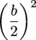. In the previous example, we added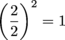. What will we add in the next example?

### Sample Problem

Solve the quadratic equation x2 – 7x + 2 = 0.

Again we have an equation we can't factor, so we need to complete the square. Using our newfangled knowledge, we know that we need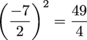. So we'll add and subtract that on the left side of our equation, to keep everything balanced.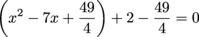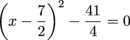When we complete the square, the term inside the parentheses is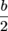, because you multiply that by itself to get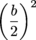in the expression you factored it from.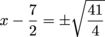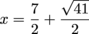and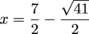We think we did everything right, but how about we double-check our results by plugging our answers back into the original equation?Ugh. UGGHH. That's a lot of fractions. Oh well, just one more to go.Whew. Unfortunately, solutions with square roots and fractions are common when dealing with quadratic equations that can't be factored.

### Sample Problem

Solve the quadratic equation 2x2 – 5x – 3 = 0 by completing the square.

This equation is easily factored: (2x + 1)(x – 3) = 0. See, we're almost done already. But the problem says we need to solve it by completing the square, so we've gotta go through with it. At least we can easily check our answer.

There's one important thing we need to point out here before continuing: completing the square will work only if a = 1. That means we need to get rid of that 2 in front of the x2 somehow. If you don't do that, terrible things will happen to you. Oh, and you'll get the problem wrong.

Let's divide the whole equation by 2.

(2x2 – 5x – 3 = 0) ÷ 2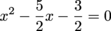Now the term we need to add or subtract is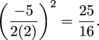That extra 2 on the bottom can be tricky, so be careful.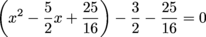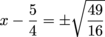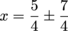That gives us two answers: x = 3 and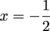.

This is exactly the result we would expect from our factored equation. If you have a choice, you want to factor if possible.

### Summing It All Up

When you're trying to complete the square, follow these steps.

• If a is anything other than 1, divide it out. You need a = 1 to even start.

• Separate the equation out into (x2 + bx) and everything else.

• Add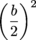to your x-values, and subtractfrom the rest.

• Factor your x-terms into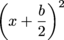oras needed.

• Isolate your squared term and take the square root of both sides.

• Simplify the expression.

The steps themselves are fairly simple, but the math can get convoluted. Like trying to play chess in your head.

Completing the square always works when solving a quadratic equation, but it's almost always a pain in the butt to actually do. We want a method that's faster and easier to use while being just as reliable.What we want is the quadratic formula. Here it is, in all its glory (when ax2 + bx + c = 0):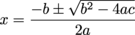You'll want to memorize this handy little formula. Luckily, it's pretty distinctive. It is possible to derive the formula from scratch, though it requires completing the square (natch). You can see the derivation here, if you like.

### Sample Problem

Solve the quadratic equation 2x2 – 5x – 3 = 0 using the quadratic formula.

We recognize this problem. We did this in the last section—there were all kinds of fractions, and it was nasty to work through. Let's see how it goes with the quadratic formula. To start, we note that a = 2, b = -5, and c = -3. We then set up the equation:### Sample Problem

Solve the equation 2x2 + 2x + 5 = 0 using the quadratic formula.

Using the quadratic formula is pretty mindless once you know the formula. Just plug and chug and plug and chug and choo choo. Sorry, we got carried away.That's right, rolling along like a train. Next we check our answers.One down, one to go.Excellent. Now we pull into the station.

• ### How Many Roots?

When you solve for the roots of a quadratic equation, there are several possible outcomes.

• You can have two real number solutions. If you set x equal to either solution, the result with be zero both times.

• There can be just one real number solution.

• The equation can have two complex number solutions. There are no real number solutions.

Don't worry; there's an easy way to find out how many solutions there are before you even start using the formula. Just take a peek at the b2 – 4ac part of the quadratic formula. That little chunk is called the discriminant, and it's the keystone species of our little quadratic ecosystem. Without it, the whole thing falls apart.

• If b2 – 4ac is positive, then there are two real number solutions.

• If b2 – 4ac = 0, then there's only one real number solution.

• If b2 – 4ac is negative, then there are two complex number solutions.

This all comes directly from the quadratic formula. If the discriminant is positive, then you have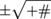, which leads to two real number answers. If it's negative, you have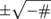, which gives two complex results. And if b2 – 4ac is 0, then you have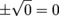, so you have only one solution.

### Sample Problem

How many roots does x2 – 3 = 0 have?

To use the discriminant, we first note that a = 1, b = 0, and c = -3.

b2 – 4ac = (0)2 – 4(1)(-3) = 12

So we have two real roots. Hah! Too easy.

How many roots does 2x2 + 8x + 8 = 0 have?

Hey now, stop it with that lip, Subheading. Why not just say "Sample Problem" like you usually do? Anyway, the discriminant for this equation is

b2 – 4ac = (8)2 – 4(2)(8) = 64 – 64 = 0

That means we have one real number root for this equation.

### How Do You Like This One, Then?

How many roots does 0.7731x2 – 2.3812x + 4.1111 = 0 have?

Now that's just being mean—but we can still do it. Just let us find our calculator real quick.

b2 – 4ac = (-2.3812)2 – 4(0.7731)(4.1111) ≈ 5.6701 – 12.7132 = -7.0431

That's negative, so there are two complex roots for this equation. Also, the calculator was in the Shmoop massage room, next to a pile of Algebra textbooks. In case you were wondering.

### What Was It Doing There?

We may have been multitasking at the time. We're pretty busy, you know.